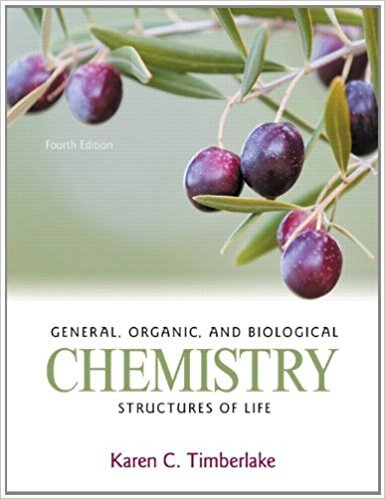# Iron(III) oxide reacts with carbon monoxide to produce## Problem 60QP Chapter 6

General, Organic, and Biological Chemistry: Structures of Life | 4th Edition

• 2901 Step-by-step solutions solved by professors and subject experts
• Get 24/7 help from StudySoup virtual teaching assistantsGeneral, Organic, and Biological Chemistry: Structures of Life | 4th Edition

4 5 0 272 Reviews
18
2
Problem 60QP

Iron(III) oxide reacts with carbon monoxide to produce iron and carbon dioxide.Fe2O3(s) + 3CO(g) ? 2Fe(s) + 3CO2(g)a. What is the percent yield for iron if the reaction of 65.0 g of iron(III) oxide produces 38.0 g of iron?b. What is the percent yield for carbon dioxide if a reaction of 75.0 g of carbon monoxide produces 85.0 g of carbon dioxide?

Step-by-Step Solution:

Solution 60QPHere, we are calculating percent yield in each of the following.Step 1 of 3Percent yield calculated by the following formulae.Percent yield (%) = 100%.____________________________________________________________________________Step 2 of 3a.Here, we are calculating the percent yield of Fe.The given chemical reactionFe2O3(s) + 3CO(g) 2Fe(s) + 3CO2(g) let’s write the equality and conversion factors for the molar mass and moles of Fe2O3 and FeMolar mass of Fe2O3 = 159.7g1 mole of Fe2O3 = 159.7 g /molSo, the conversion factors are and Molar mass of Fe = 55.9 g1 mole of Fe = 55.9 g/molSo, the conversion factors are and From the given chemical equation Mole-mole factors of Fe and Fe2O3 are1 mol of Fe2O3 = 2 mol of FeSo, the conversion factors are and Let’s calculate the Fe produced by using 65.0 g of Fe2O365.0 g Fe2O3 = 45.5 g of Fe.Therefore, theoretical yield of Fe is 45.5g.Let’s calculate the percent yield of Fe.Percent yield (%) = 100%.Actual yield of Fe - 38.0gTheoretical yield - 45.5gPercent yield = 100% = 83.5%Therefore, percent yield of Fe is 83.5%.______________________________________________________________________________

Step 3 of 3

##### ISBN: 9780321750891

×
Get Full Access to General, Organic, And Biological Chemistry: Structures Of Life - 4 Edition - Chapter 6 - Problem 60qp

Get Full Access to General, Organic, And Biological Chemistry: Structures Of Life - 4 Edition - Chapter 6 - Problem 60qp

I don't want to reset my password

Need help? Contact support

Need an Account? Is not associated with an account
We're here to help